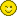# Calculating the area of any quadrilateral

Every quadrilateral is a polygon with four sides of any length connected together at the corners. The method of calculation for quadrilaterals is triangulation, which requires you to know the lengths of one of the two diagonals.

Through this formula, it's possible to find out the area of any quadrilateral, no matter if it's a parallelogram, rhombus, trapezoid – in short, every 4-sided surface expect a crossed quadrilateral.

Note: this form uses the triangulation calculation method.

* Be careful to respect the order of the sides and use the diagonal 'ab' as shown on the drawing, otherwise you may get a wrong result.

Units:
Side a:
Side b:
diagonal ab:
Side c:
Side d:
Decimal places:
Units angles:
 Surface: 0,87 m2 Perimeter: 4,00 m Quadrilateral angles: (alfa) α : 60,00 ° (beta) β : 120,00 ° (gama) γ : 60,00 ° (delta) δ : 120,00 °

## The Angles of a Quadrilateral

The calculation is done as follows: find the sum of the squares of the two adjacent sides of the angle, which is then subtracted from the square of the opposite diagonal. Then, divide the result of this "addition/subtraction" by the product of the two adjacent sides times two. Finally, you calculate the arccosine of the above result. What a cinch!Now for those who need it, you can find aspirin, Tylenol or other headache medicine at your nearest drug store…

Knowing the calculation of any quadrilateral is useful for determining the area of household objects with precision – even more so in older homes. It's common to have rooms in which the walls are not perpendicular with each other, making them not just rectangles, but various types of quadrilaterals.

All the same, calculating the land surface area is very difficult if the surface is considered a rectangle, which is hardly ever the case. By knowing its four sides, one of its two diagonals and using the formula on this page, your results will be spot-on.